Function Repository Resource:

RandomWolframModel

Generate a random canonical Wolfram model rule with a particular signature

Contributed by: Stephen Wolfram & Max Piskunov
 ResourceFunction["RandomWolframModel"][signature] generates a random Wolfram model rule with the specified signature. ResourceFunction["RandomWolframModel"][signature,n] generates a random Wolfram model using at most n distinct elements.

Details and Options

The signature is given in the form lhsrhs, where each lhs or rhs is a list of pairs {count,arity} where count gives the number of hyperedges of specified arity.
ResourceFunction["RandomWolframModel"] gives a canonical form of each rule it returns.

Examples

Basic Examples (3)

Generate a random 23 32 Wolfram model rule:

 In:=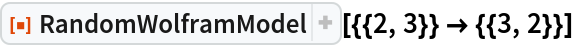Out=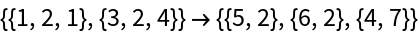Generate a 23 32 Wolfram model rule, restricting to two distinct elements:

 In:=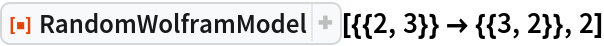Out=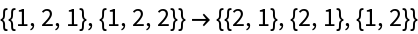Generate a random 23 32 Wolfram model rule:

 In:=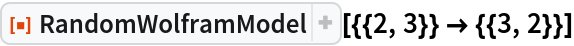Out=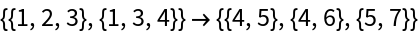Generate a random 23224382 with at most 12 elements:

 In:=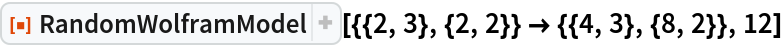Out=Generate a random rule containing two different transformations:

 In:=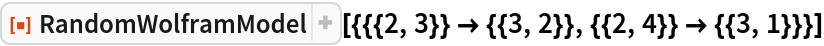Out=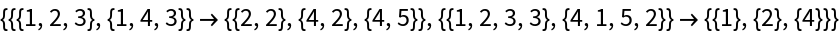Possible Issues (2)

By default (Automatic connectedness), disconnected right-hand sides can be generated as long as all of their components are still connected to the left-hand side:

 In:=Out=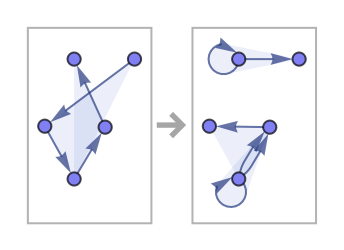Note that evolution may still be connected in this case if there are other edges connecting vertices on the left-hand side:

 In:=Out=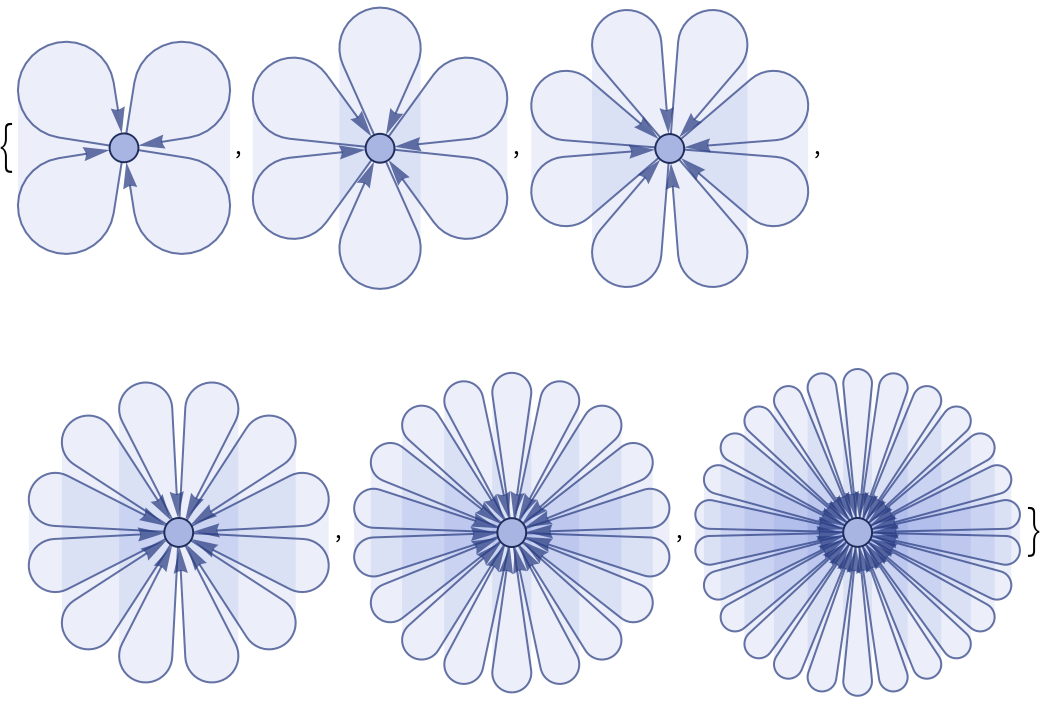Author Notes

In its present form, this will not correctly weight all canonical forms the same.